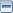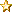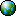January 26, 2021, 12:20:01 pmДобре дошъл/дошла, Гост. Моля, въведи своето потребителско име или се регистрирай.Изгуби ли регистрационния е-мейл? 1 Час 1 Ден 1 Седмица 1 Месец Завинаги Влез с потребителско име, парола и продължителност на сесията
 Новини: SMF - Just Installed!Заглавна Страница Помощ Търси Календар Вход Регистрирай
 Страници:Автор Тема: Това нормално ли е ?  (Прочетена 5452 пъти) 0 Членове и 1 Гост преглежда(т) тази тема.
stancho
Sr. MemberПубликации: 455« -: January 26, 2009, 04:05:46 pm »

Здравейте от 2 дни нета много се влачи и искам да ми кажете дали това е нормално при положение, че сега е 3 наобяд и не би следвало да има голямо натоварване.

Pinging www.l.google.com [74.125.77.103] with 32 bytes of data:

Reply from 74.125.77.103: bytes=32 time=69ms TTL=0
Reply from 74.125.77.103: bytes=32 time=70ms TTL=0
Reply from 74.125.77.103: bytes=32 time=69ms TTL=0
Reply from 74.125.77.103: bytes=32 time=69ms TTL=0
Reply from 74.125.77.103: bytes=32 time=69ms TTL=0
Reply from 74.125.77.103: bytes=32 time=69ms TTL=0
Reply from 74.125.77.103: bytes=32 time=71ms TTL=0
Reply from 74.125.77.103: bytes=32 time=69ms TTL=0
Reply from 74.125.77.103: bytes=32 time=70ms TTL=0
Request timed out.
Reply from 74.125.77.103: bytes=32 time=70ms TTL=0
Reply from 74.125.77.103: bytes=32 time=69ms TTL=0
Reply from 74.125.77.103: bytes=32 time=69ms TTL=0
Reply from 74.125.77.103: bytes=32 time=70ms TTL=0
Reply from 74.125.77.103: bytes=32 time=69ms TTL=0
Reply from 74.125.77.103: bytes=32 time=69ms TTL=0
Reply from 74.125.77.103: bytes=32 time=69ms TTL=0
Request timed out.
Reply from 74.125.77.103: bytes=32 time=70ms TTL=0
Request timed out.
Reply from 74.125.77.103: bytes=32 time=70ms TTL=0
Reply from 74.125.77.103: bytes=32 time=71ms TTL=0
Request timed out.
Reply from 74.125.77.103: bytes=32 time=70ms TTL=0
Request timed out.
Reply from 74.125.77.103: bytes=32 time=69ms TTL=0
Reply from 74.125.77.103: bytes=32 time=69ms TTL=0
Reply from 74.125.77.103: bytes=32 time=70ms TTL=0
Request timed out.
Reply from 74.125.77.103: bytes=32 time=70ms TTL=0
Reply from 74.125.77.103: bytes=32 time=70ms TTL=0
Reply from 74.125.77.103: bytes=32 time=72ms TTL=0
Request timed out.
Request timed out.
Reply from 74.125.77.103: bytes=32 time=70ms TTL=0
Request timed out.
Request timed out.
Reply from 74.125.77.103: bytes=32 time=70ms TTL=0
Reply from 74.125.77.103: bytes=32 time=69ms TTL=0
Request timed out.
Reply from 74.125.77.103: bytes=32 time=69ms TTL=0
Reply from 74.125.77.103: bytes=32 time=70ms TTL=0
Request timed out.
Reply from 74.125.77.103: bytes=32 time=70ms TTL=0
Reply from 74.125.77.103: bytes=32 time=70ms TTL=0
Reply from 74.125.77.103: bytes=32 time=71ms TTL=0
Request timed out.
Reply from 74.125.77.103: bytes=32 time=70ms TTL=0
Reply from 74.125.77.103: bytes=32 time=71ms TTL=0
Reply from 74.125.77.103: bytes=32 time=70ms TTL=0
Request timed out.
Reply from 74.125.77.103: bytes=32 time=70ms TTL=0
Reply from 74.125.77.103: bytes=32 time=70ms TTL=0
Reply from 74.125.77.103: bytes=32 time=70ms TTL=0
Request timed out.

Ping statistics for 74.125.77.103:
Packets: Sent = 55, Received = 40, Lost = 15 (27% loss),
Approximate round trip times in milli-seconds:
Minimum = 69ms, Maximum = 72ms, Average = 69msАктивен
hipodruma
NewbieПубликации: 36« Отговор #1 -: January 26, 2009, 05:30:46 pm »

http://forum.optic-com.eu/index.php/topic,717.0.htmlАктивен
 Страници: 
Отиди на:

Страницата е създадена за 0.109 секунди с 18 запитвания.